# Some stuff I learned this week

This week I had the pleasure to spend 3 days at a wonderful workshop (disclaimer: I was part of the organization, with Ofer Dekel, Yuval Peres and James Lee, so I might be a bit biased). Below you will find three results that I particularly enjoyed (one from each day). Obviously many more topics were covered at the workshop, from finding correlations in subquadratic time, to the basic problem of learning halfspaces (see for instances these two COLT’15 papers, by Daniely and by Awasthi, Balcan, Haghtalab and Urner), or how stability can explain why deep nets trained with SGD are not overfitting (upcoming work by Hardt-Recht-Singer; also on a related note I learned from Yuval about the Kolmogrov-Arnold representation theorem, which seems very much related to the famous Barron’1990 result on the universal approximation properties of neural networks with 1 hidden layer). Finally two more things: (i) we also have a blog where we collected all the open problems discussed during the workshop, and (ii) many of the talks have been recorded (including the ones I mention below, as well as the nice tutorial by Sergiu Hart on dynamics in games). I will post the link to the videos as soon as we upload them.

Negative association

Robin Pemantle gave an excellent tutorial on negative association, see the slides here. The punchline can be summarized pretty succinctly. Consider a measureon. We say thatis positively correlated if for anymonotone increasing one has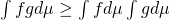. On the other hand we say thatis negatively correlated if for anymonotone increasing and such thatand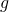depends on disjoint subset of variables, one has. Positive correlation is particularly easy to check thanks to the famous FKG theorem which states that ifis log-supermodular (i.e.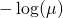is a submodular function) thenis positively correlated (this is nice because submodularity is a local condition). One may hope that log-submodular distributions are negatively correlated, but unfortunately this is not true in general. The route to an easily checkable condition for negative correlation is a bit more tortuous and it goes as follows. First observe that negative association clearly implies pairwise negative correlation, that is for any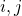and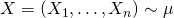,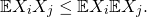Writing this inequality in terms of the generating function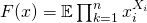one has (with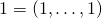):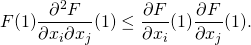One says thatis strongly Rayleigh if the above equation holds true for any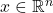instead of just at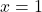. It turns out that this condition is more restrictive than negative correlation, but it is also much more tractable, in particular because it is equivalent to the fact thatis a stable polynomial (that isdoes not have any roots in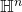whereis the complex upper half plane). Once you know that latter equivalence it becomes trivial to check that determinantal measures are negatively correlated since they are strongly Rayleigh (just use the fact that the characteristic polynomial of a symmetric matrix only has roots on the real line). A particularly useful property of strongly Rayleigh measures is that they have the usual Gaussian tails for any Lipschitz functional, just like product measures (this was conjectured by Elchanan Mossel, and recently proved by Robin Pemantle and Yuval Peres). Recently this set of measures has also been useful in theoretical computer science, see the recent work of Shayan Oveis Gharan and his co-authors (where they use negative correlation properties of random spanning trees). Finally I briefly mention that the sampling problem for negatively associated measures is a quite rich question, see the slides by Robin and also this COLT’15 paper by Rebeschini and Karbasi.

Learning in networks

Elchanan Mossel talked about a beautiful set of open questions. The basic problem goes as follows: letbe a graph, and assume that each vertex is a player that receives an independent random variable distributed according to some measure. The measure used to produce the random variables is the same for all vertices, and it is either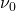or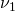(say the players also know that the choice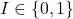of the measure was a coin flip). Question: assuming that at the end of each day the players reveal their conditional expectation for(given all the information they currently have) to their neighbors in, how many days do we need to reach an agreement? If convergence happens, is the conditional expectation that is reached the optimal one (that is the conditional expectation given all the random variables)? What if instead of revealing your belief you only reveal what is the most likely to you between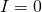and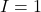(revealed action game)? Mossel-Sly-Tamuz show that both in the revealed belief and the revealed action game there will eventually be an agreement. Furthermore in the revealed beliefs this will be close to the optimal posterior provided thatis large enough. Both of these theorems currently lack a convergence rate.

The complexity of stochastic block models

The stochastic block model is a canonical model of a random graph with communities. The general version of this model is denotedwhereis the number of vertices,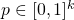represents the proportion of each community, and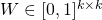represents the matrix of connection probabilities. More precisely the random graphis defined as follows: First each vertexis assigned a latent label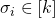, where the latent label vectoris drawn uniformly at random conditionally on the fact that the number of vertices with label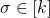is exactly. The edges are then drawn independently (conditionally on), and each edge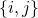is present with probability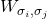. This model has received a LOT of attention in the last thirty years. The basic objective is to recover the latent label vectorfrom the observation of the unlabelled graph. The following very recent result by Emmanuel Abbe and Colin Sandon answers the most basic question one can ask: when is this objective achievable? First we introduce the Abbe-Sandon divergence for vectors: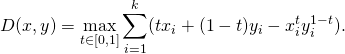Theorem (Abbe-Sandon 2015): Asymptotically almost sure exact recovery of the latent feature vector in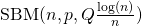is possible if and only if for any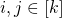,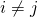,In their paper Abbe and Sandon also give an efficient algorithm which achieves the information theoretic limit given above. It remains an outstanding open problem to obtain such a precise picture for the problem of weak recovery where one asks to recover correctly only a fraction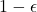of the latent label vector. This question is particularly interesting in the sparse regime where the average degree is constant (note that in the above theorem the average degree diverges at the rate). Recently a lot of progress has been made in this mathematically challenging regime too, see in particular the papers by Mossel-Neeman-Sly, Massoulie and Bordenave-Lelarge-Massoulie.

This entry was posted in Conference/workshop, Probability theory. Bookmark the permalink.